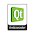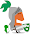## Thursday, November 17, 2011

### Virtual Joystick with QML

Recently out of curiosity I tried to created virtual joystick, which nowadayx s used quite frequently touch based games.

Well my implementation still required some improvement to provide real feel of joystick but its still quite useful. Here is output.

To test my joystick, I also created a sprite which moves in almost all direction.

Here is my QML code for joystick. I basically used two circle and checking inner circle position with outer circle and deciding direction. I am not describing code, but tried to put necessary comment in code.
```import QtQuick 1.0

Item {
id:joyStick;
property int offset:30;

signal dirChanged(string direction);
signal pressed();
signal released();

Rectangle {
id:totalArea
color:"gray"
opacity: 0.5
width:parent.width;height:parent.height
}

Rectangle{
id:stick
width:totalArea.width/2; height: width
x: totalArea.width/2 - radius;
y: totalArea.height/2 - radius;
color:"black"
}

MouseArea{
id:mouseArea
anchors.fill: parent

onPressed: {
joyStick.pressed();
}

onMousePositionChanged: {
//(x-center_x)^2 + (y - center_y)^2 < radius^2
//if stick need to remain inside larger circle
//if stick can go outside larger circle

// calculate distance in x direction
var xDist = mouseX - (totalArea.x + totalArea.radius);
xDist = xDist * xDist;

// calculate distance in y direction
var yDist = mouseY - (totalArea.y + totalArea.radius);
yDist = yDist * yDist;

//total distance for inner circle
var dist = xDist + yDist;

//if distance if less then radius then inner circle is inside larger circle
if( rad < dist) {
return;
}

//center of larger circle
var oldX = stick.x; var oldY = stick.y;
stick.x = mouseX - stick.radius;
stick.y = mouseY - stick.radius;

//using L R U D LU RU LD RD for describe direction
var dir="";

//check if Right or left direction,
//by checking if inner circle's y is near center of larger circle
if( stick.y >= totalArea.radius - stick.radius - joyStick.offset
&& stick.y+stick.height <= totalArea.radius + stick.radius + joyStick.offset) {
if( stick.x + stick.radius > totalArea.x + totalArea.radius) {
dir = "R";
} else if( stick.x < totalArea.x + totalArea.radius) {
dir = "L";
}
}
//check if Up or Down direction,
//by checking if inner circle's x is near center of larger circle
else if( stick.x >= totalArea.radius - stick.radius - joyStick.offset
&& stick.x + stick.width <= totalArea.radius + stick.radius + joyStick.offset) {
if( stick.y + stick.radius > totalArea.y + totalArea.radius) {
dir = "D";
} else if( stick.y < totalArea.y + totalArea.radius) {
dir = "U";
}
}
//check if Up Left or Up Right direction,
//by checking if inner circle is near one of top corner of larger circle
else if( stick.y < totalArea.radius - stick.radius ) {
if( stick.x + stick.radius > totalArea.x + totalArea.radius) {
dir = "R";
} else if( stick.x < totalArea.x + totalArea.radius) {
dir = "L";
}
dir = dir +"U";
}
//check if Down Left or Down Right direction,
//by checking if inner circle is near one of bottom corner of larger circle
else if ( stick.y + stick.radius >= totalArea.radius + stick.radius ) {
if( stick.x + stick.radius > totalArea.x + totalArea.radius) {
dir = "R";
} else if( stick.x < totalArea.x + totalArea.radius) {
dir = "L";
}
dir = dir +"D";
}

joyStick.dirChanged(dir);
}

onReleased: {
//snap to center
stick.x = totalArea.width /2 - stick.radius;
stick.y = totalArea.height/2 - stick.radius;

joyStick.released();
}
}
}

```
Follwing my code for sprite used in above video.
```import QtQuick 1.0

Item {
id:sprite
width: 50; height: 50
clip:true
property alias running: timer.running;
property int frameCount: 5
property int frame:0
property int row:0

property int xDir:0;
property int yDir:0;

Image{
id:image
source:"man.png"
x:-sprite.width * sprite.frame;
y:-sprite.height * sprite.row;
}

function changeDirection(direction) {
if( direction === "L") {
sprite.row=6;
sprite.xDir = -1;
sprite.yDir = 0;
} else if( direction === "R") {
sprite.row=2;
sprite.xDir = 1;
sprite.yDir = 0;
} else if( direction === "U") {
sprite.row=4;
sprite.xDir = 0;
sprite.yDir = -1;
} else if( direction === "D") {
sprite.row=0;
sprite.xDir = 0;
sprite.yDir = 1;
} else if( direction === "RU") {
sprite.row = 3;
sprite.xDir = 1;
sprite.yDir = -1;
} else if( direction === "LU") {
sprite.row = 5;
sprite.xDir = -1;
sprite.yDir = -1;
} else if( direction === "RD") {
sprite.row = 1;
sprite.xDir = 1;
sprite.yDir = 1;
} else if( direction === "LD") {
sprite.row = 7;
sprite.xDir = -1;
sprite.yDir = 1;
}
}

function nextFrame() {
sprite.frame = ++sprite.frame  % sprite.frameCount
sprite.x = sprite.x + 5* sprite.xDir;
sprite.y = sprite.y + 5* sprite.yDir;
}

Timer {
id:timer
interval: 150; running: false; repeat: true
onTriggered: {
nextFrame();
}
}
}
```
Finally, main qml file which include both joystick and sprite.
```import QtQuick 1.0

Image {
width: 854 ;  height:480
source:"grass.jpg"
fillMode: Image.Tile

Sprite{
id:man
x:parent.width/2 ; y: parent.height/2;
}

Connections {
target: joyStick
onDirChanged:{
man.changeDirection(direction)
}

onPressed:{
man.running=true;
}

onReleased:{
man.running=false;
}
}

Joystick{
id:joyStick
anchors.bottom: parent.bottom
anchors.left: parent.left
anchors.bottomMargin: 10
anchors.leftMargin: 10
width:150;height:150
}
}

```

1.Very nice!
The video is too good!
But, I am not able to implement the sprites in my project.

Can you please provide the project? It will really help!

Brilliant stuff that I used to see only some TOP companies doing it! But, V-Joystick is too good!

Thank You.

2.Hi,
Thanks for your comment, glad that you liked it.

I will upload code soon and post link here.

Thanks and regards,
Kunal

3.hey kunal parmar this is a great project (y) i wander if u can give the full source :/ of this great app that u creat :( !!!!

1.Sure, it must be lying somewhere but I need to find the original source now. will try to find it.